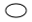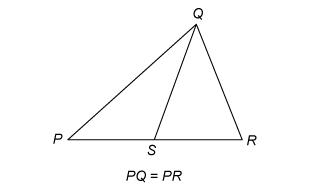# GRE Quantitative Reasoning Questions

100 Quantitance Comparison Questions

 Free Online Vocabulary TestK12, SAT, GRE, IELTS, TOEFL
 Select Exercise Lists

By Question Type

By Content

 Selected Set: 1
Select Exercise Set:

Select Question:

1.

 Quantity A Quantity B The least prime number greater than 24 The greatest prime number less than 28Quantity A is greater.Quantity B is greater.The two quantities are equal.The relationship cannot be determined from the information given.
2.

 Lionel is younger than Maria. Quantity A Quantity B Twice Lionel's age Maria's ageQuantity A is greater.Quantity B is greater.The two quantities are equal.The relationship cannot be determined from the information given.
3.

 Quantity A Quantity B 54% of 360 150Quantity A is greater.Quantity B is greater.The two quantities are equal.The relationship cannot be determined from the information given.
4.Quantity A Quantity B PS SRQuantity A is greater.Quantity B is greater.The two quantities are equal.The relationship cannot be determined from the information given.
5.

 y=2x2+7x-3 Quantity A Quantity B x yQuantity A is greater.Quantity B is greater.The two quantities are equal.The relationship cannot be determined from the information given.
6.

 y>4 Quantity A Quantity B (3y+2)/5 7Quantity A is greater.Quantity B is greater.The two quantities are equal.The relationship cannot be determined from the information given.
7.

 Quantity A Quantity B (230 - 229)/2 228Quantity A is greater.Quantity B is greater.The two quantities are equal.The relationship cannot be determined from the information given.
8.

 Quantity A Quantity B x2+1 2x-1Quantity A is greater.Quantity B is greater.The two quantities are equal.The relationship cannot be determined from the information given.
9.

 w>1 Quantity A Quantity B 7w-4 2w+5Quantity A is greater.Quantity B is greater.The two quantities are equal.The relationship cannot be determined from the information given.
10.

 Quantity A Quantity B (-3)7 (-3)6Quantity A is greater.Quantity B is greater.The two quantities are equal.The relationship cannot be determined from the information given.